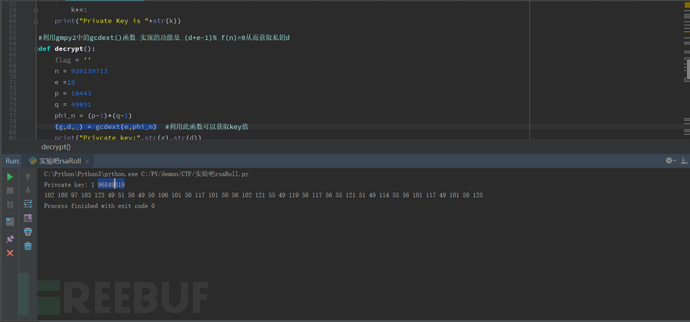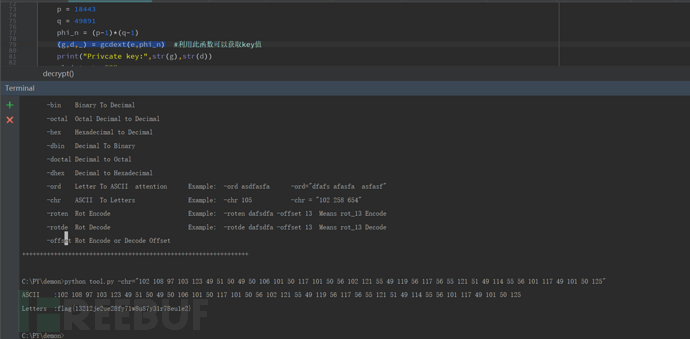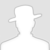FreeBuf.COM网络安全行业门户，每日发布专业的安全资讯、技术剖析。FreeBuf+小程序

2018-04-12 10:00:48

## 0x1

（1）选择一对不同的、足够大的素数p，q。

（2）计算n=pq。

（3）计算f(n)=(p-1)(q-1)，同时对p, q严加保密，不让任何人知道。

（4）找一个与f(n)互质的数e，且1<e<f(n)。

（5）计算d，使得de≡1 modf(n)。这个公式也可以表达为(d*e-1)% f(n)=0

这里要解释一下，≡是数论中表示同余的符号。公式中，≡符号的左边必须和符号右边同余，也就是两边模运算结果相同。

显而易见，不管f(n)取什么值，符号 右边1 mod f(n)的结果都等于1；符号的左边d与e的乘积做模运算后的结果也必须等于1。

这就需要计算出d的值，让这个同余等式能够成立。

（6）公钥KU=(e,n)，私钥KR=(d,n)。

（7）加密时，先将明文变换成0至n-1的一个整数M。若明文较长，可先分割成适当的组，然后再进行交换。设密文为C，

则加密过程为：C≡M^e(mod n)。

（8）解密过程为：M≡C^d(mod n)。

p: 第一个大素数

q: 第二个大素数

f(n):  (p-1)*(q-1)

## 0x2

http://ctf5.shiyanbar.com/crypto/RSAROLL.txt

{920139713,19}

(1)   n = 920139713  e =19

(2)   这时候我们要将整数n分解为两个素数，我们可以使用在线网站直接来获取。

http://www.factordb.com/index.php

``如下：defdivde(n):        start =2        whilestart < math.sqrt(n):        if (n%start==0):            record = start            print(“大整数可以分解为:”,str(start),str(int(n/base)))            break         base+=1         return (start,int(n/base))``

(3)   经过上面的操作我们可以获得

p = 18443,q =49891,f(n) = (p-1)*(q-1)/p，q 是上n分解的两个质数，f(n)是欧拉函数

(4)   根据我们现在已经获得的条件以及前面补充的RSA知识我们知道只要找到一个满足e * d ≡ 1 (mod f(n)) 这个方程式的d，我们就成功获取的私钥(于是我就自己写了一个函数跑了一下，跑了好长时间仍然没有结果，在我快要放弃的时候我忽然想到了gmpy2，其中有一个神奇的函数)

(g,d,_) = gcdext(e,f(n)) //不知道为什么是这种格式但是它的效果是与e * d ≡ 1 (mod f(n)) 使用此函数只用了不到一秒的时间就获得了私钥d= 96849619

(5)   至此成功拿到了私钥，剩下的就剩解密密文(6)   最后将ASCII转化为相应的字符 (这里我用了自己写的一个小脚本工具)## 0x3代码部分

``#-*- coding:utf-8 -*-from gmpy2 import *from Crypto.Util.number import *利用gmpy2中的gcdext()函数实现的功能是 (d*e-1)% f(n)=0从而获取私钥d def decrypt():   flag = ''    n= 920139713    e=19    p= 18443    q= 49891   phi_n = (p-1)*(q-1)   (g,d,_) = gcdext(e,phi_n)  #利用此函数可以获取私钥d值   print("Privcate key:",str(g),str(d))   plaintext =""" 704796792                    752211152                    274704164                    18414022                    368270835                    483295235                    263072905                    459788476                    483295235                    459788476                    663551792                    475206804                    459788476                    428313374                    475206804                    459788476                    425392137                    704796792                    458265677                    341524652                    483295235                    534149509                    425392137                    428313374                    425392137                    341524652                    458265677                    263072905                    483295235                    828509797                    341524652                    425392137                    475206804                    428313374                    483295235                    475206804                    459788476                    306220148   """    plaintext = plaintext.split()   for i in plaintext:       #利用pow(l,d,n) l的d次方然后取n的余数       i = int(i)       print((pow(i,d,n)),end=' ')if __name__=='__main__':  decrypt()``

## 0x4 后记

*本文作者：白帽子马振华，转载请注明FreeBuf.COM

# RSA破解 # 加密 # 解密

+ 收入我的专辑• 0 文章数
• 0 评论数
• 0 关注者